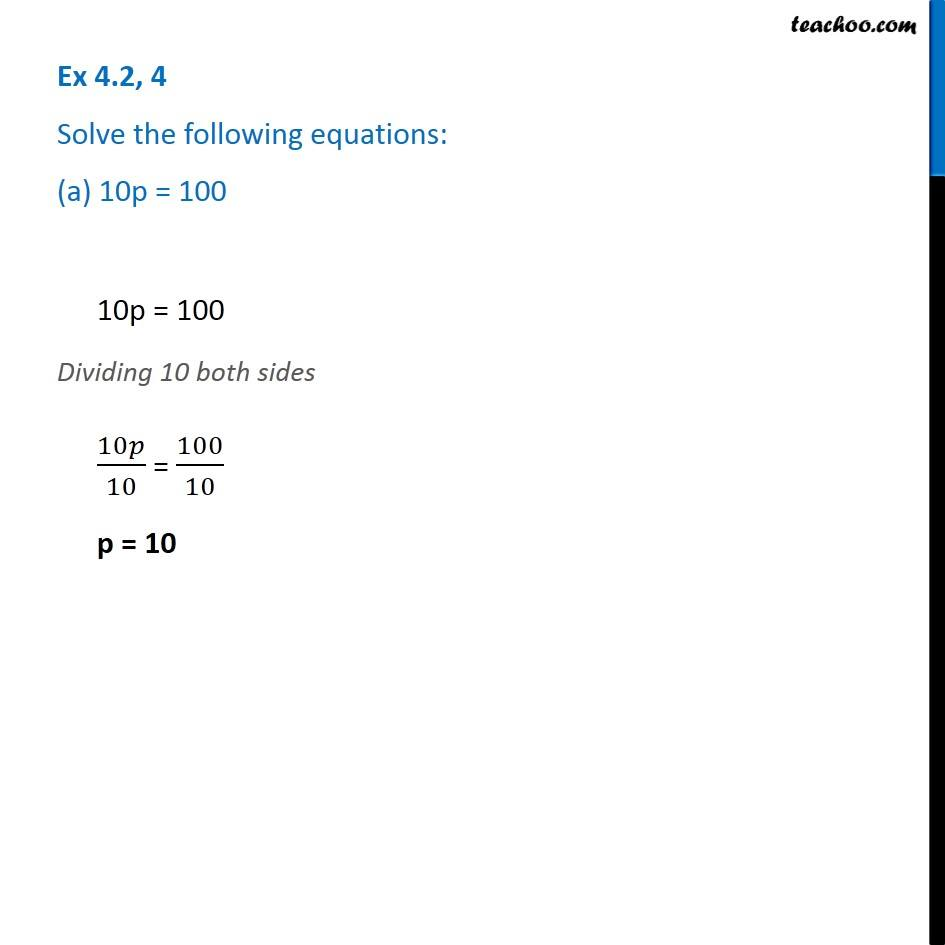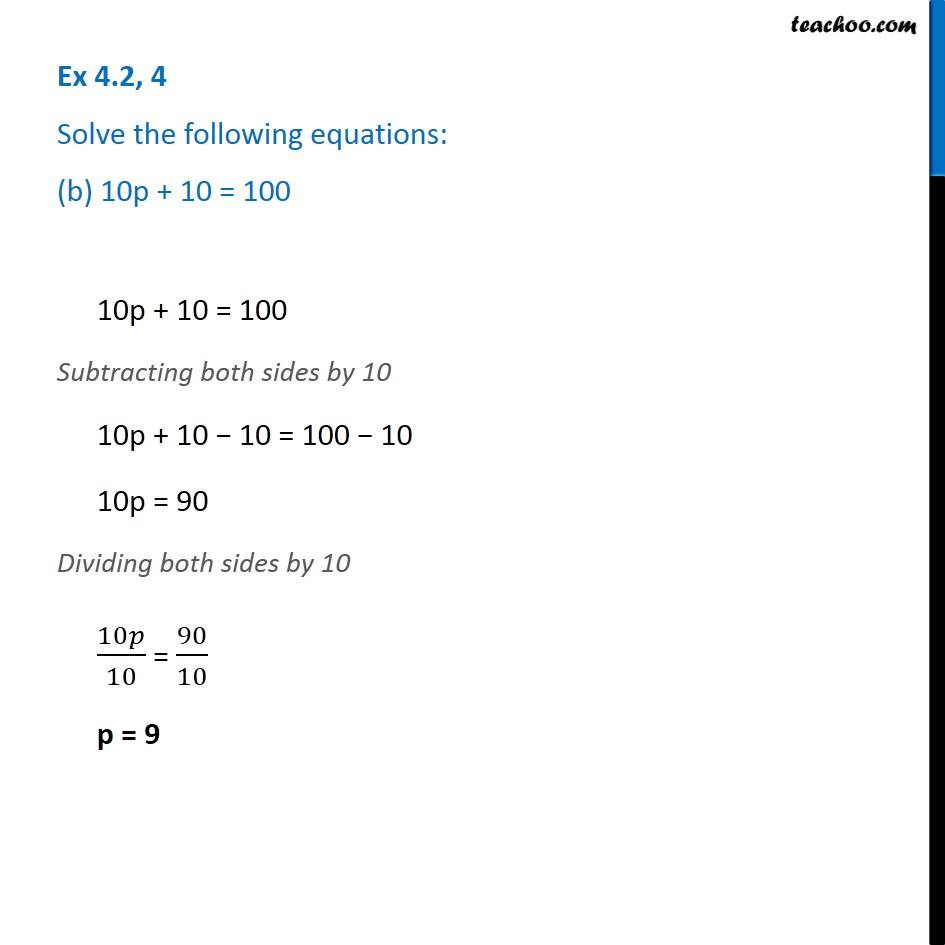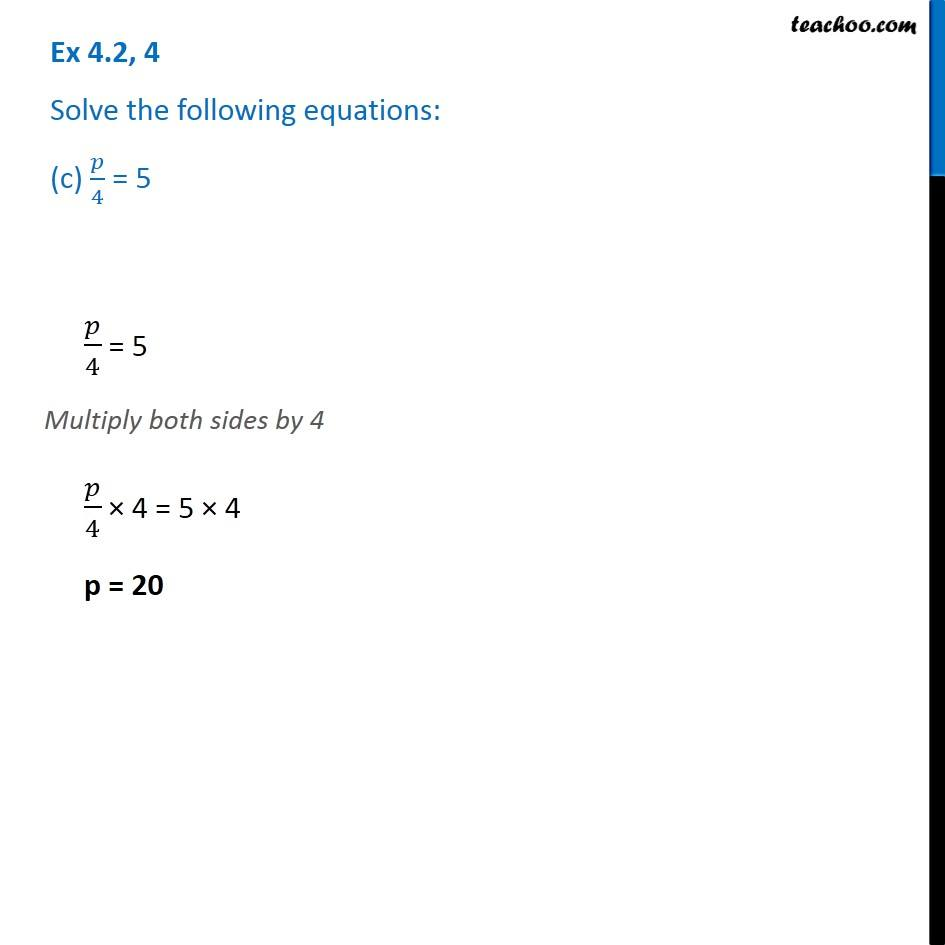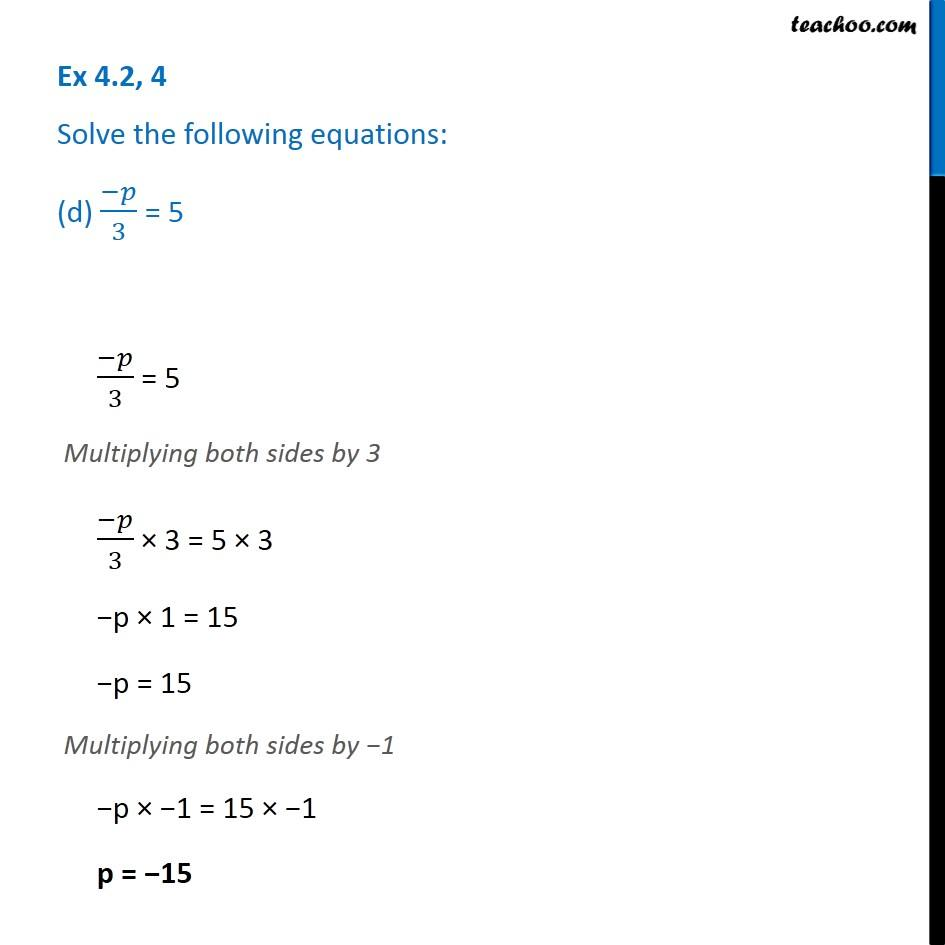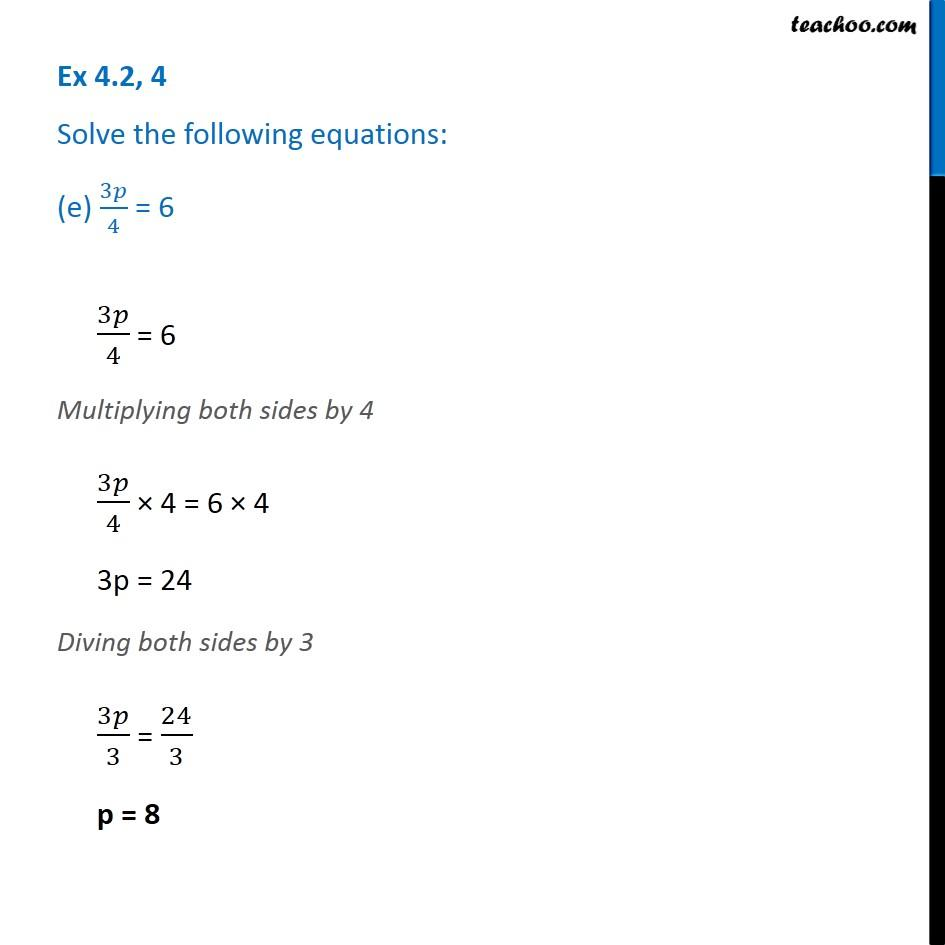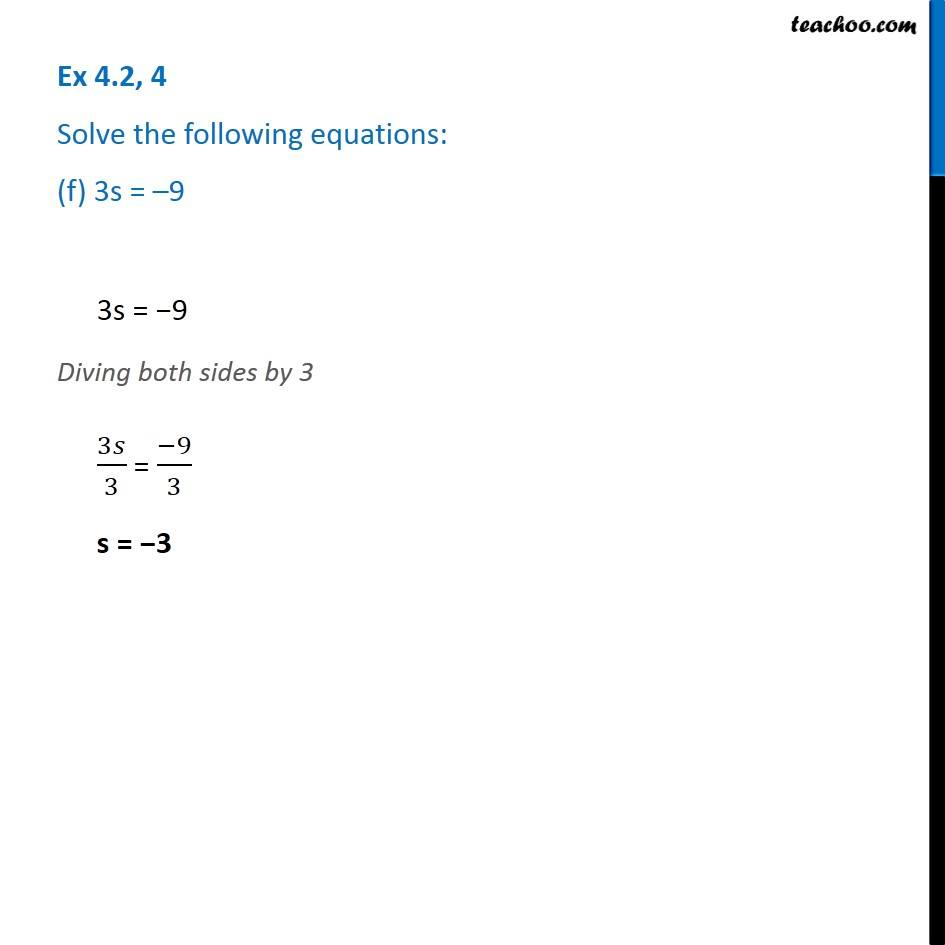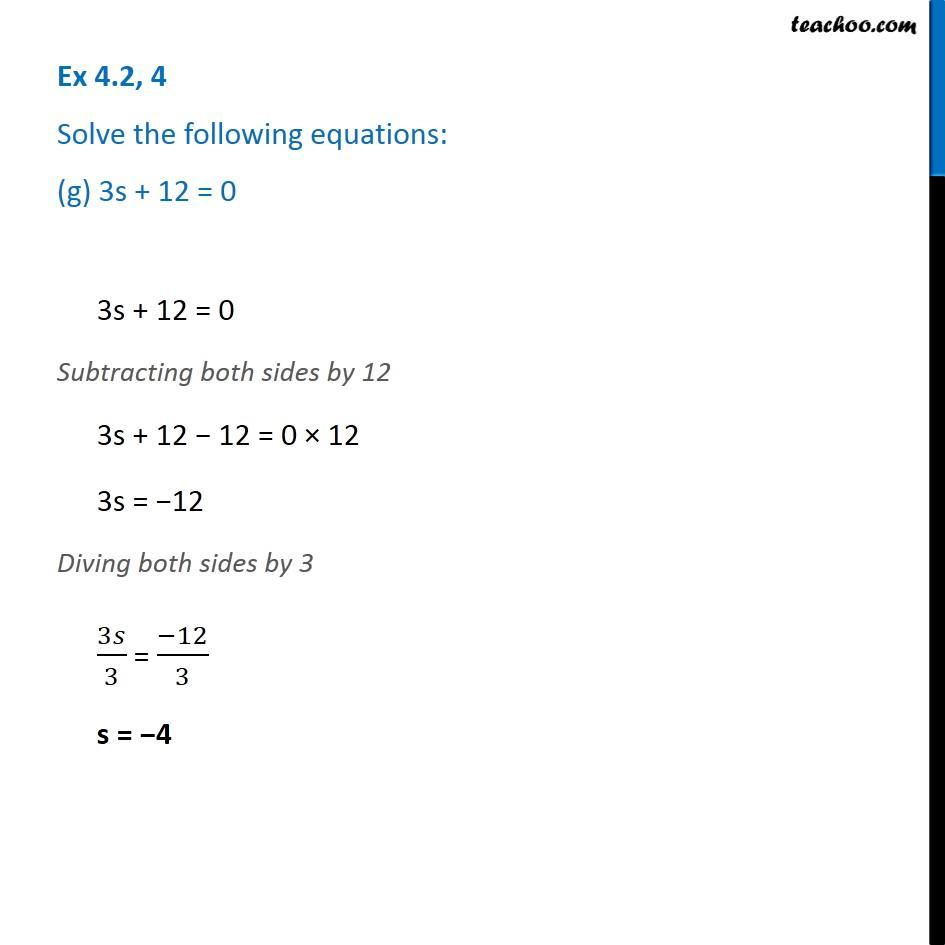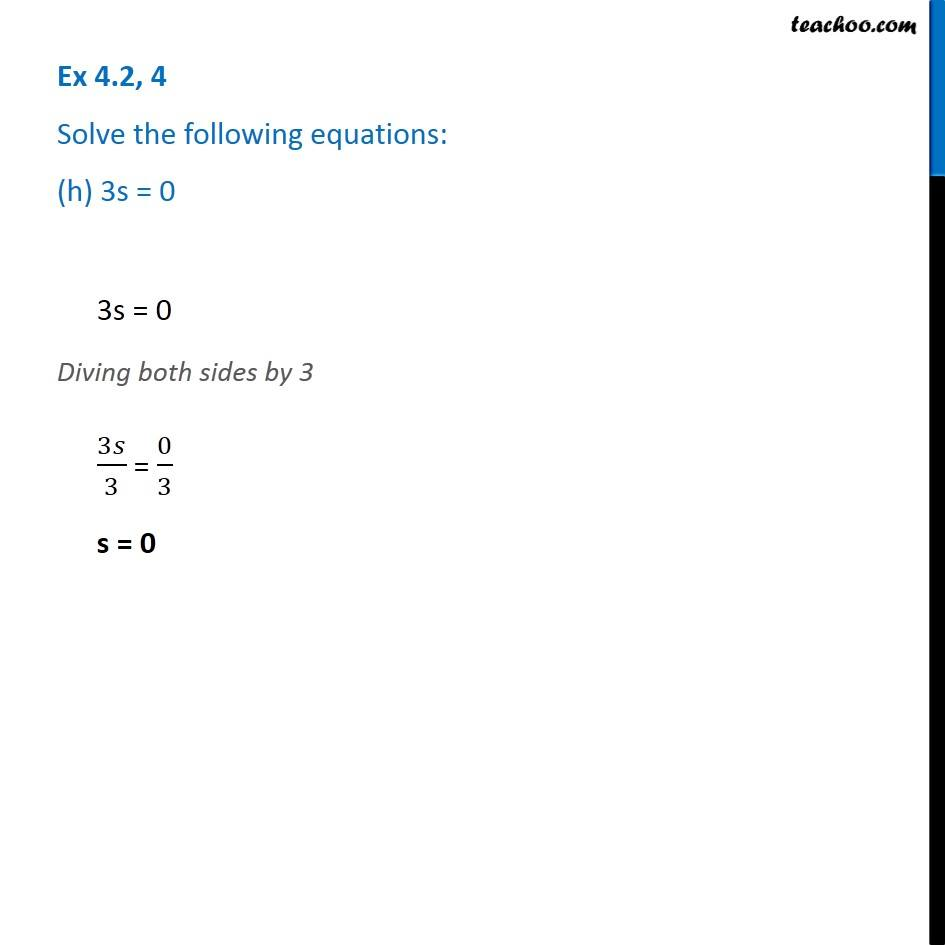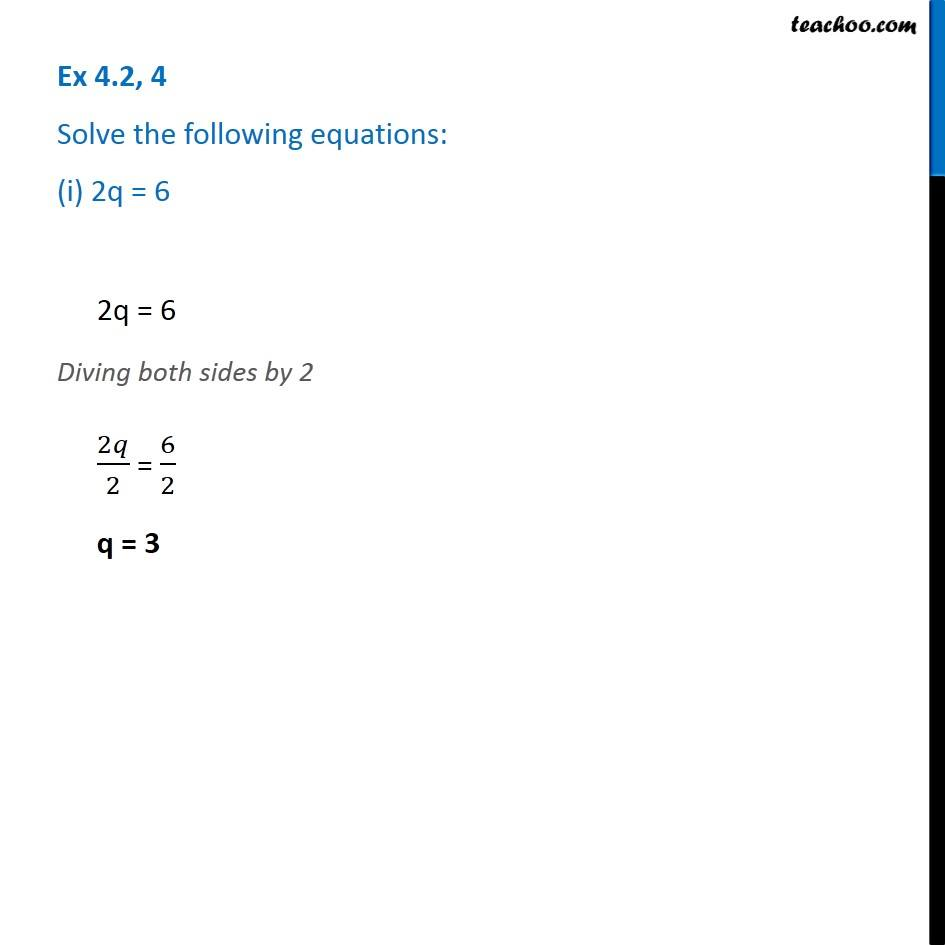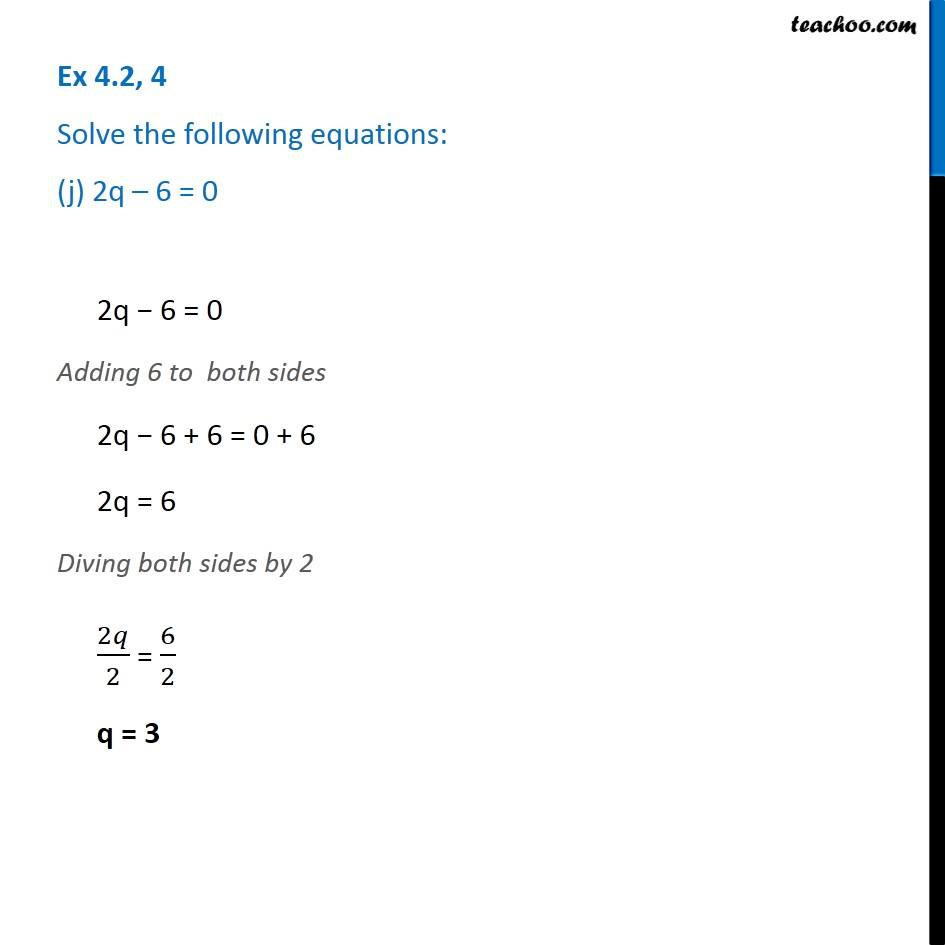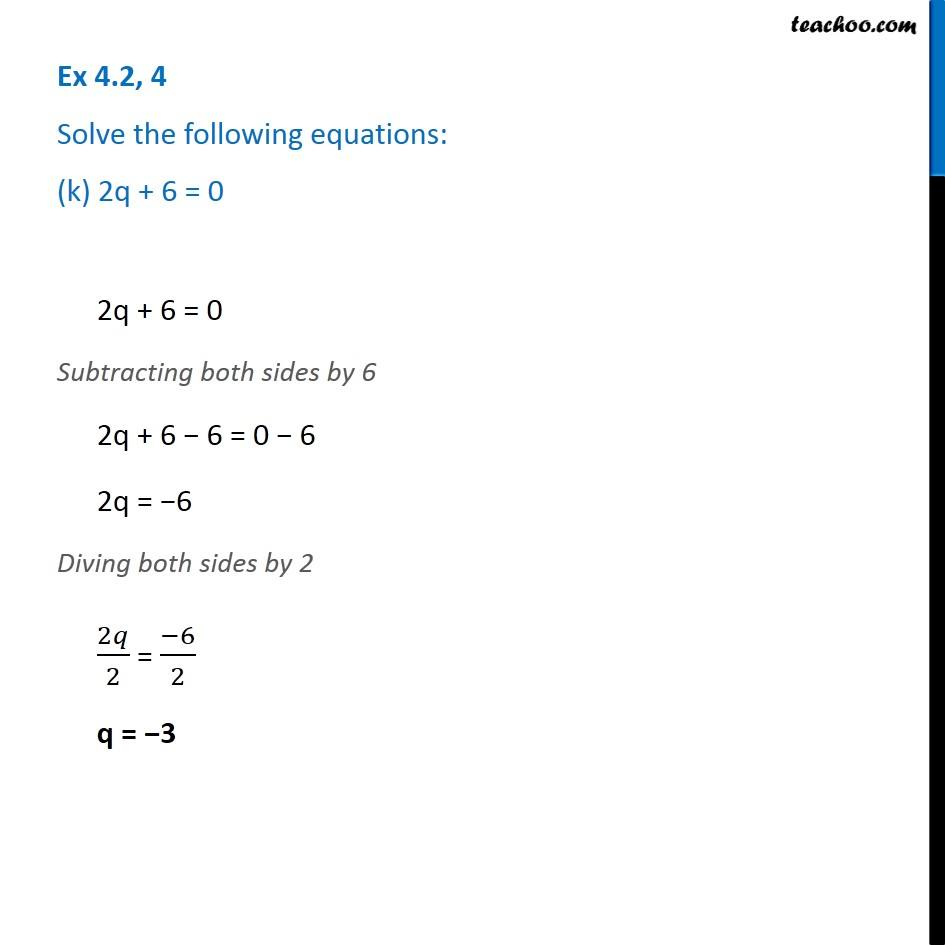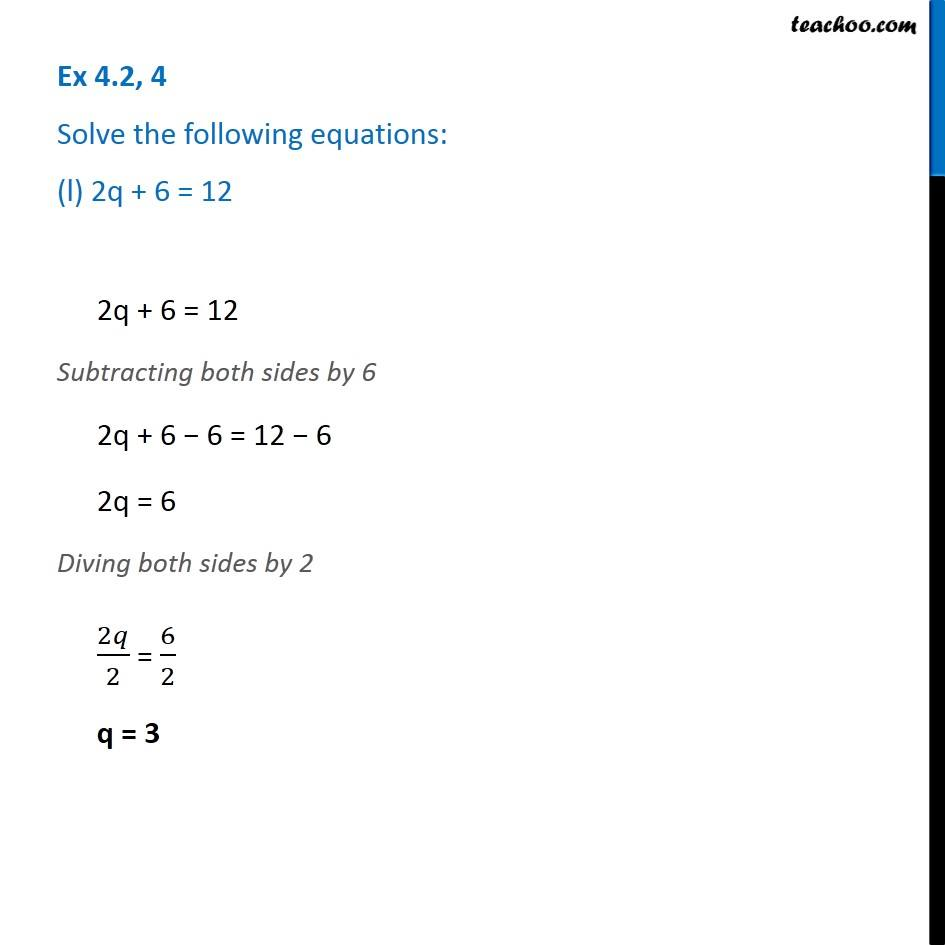Subscribe to our Youtube Channel - https://you.tube/teachoo

1. Chapter 4 Class 7 Simple Equations
2. Serial order wise
3. Ex 4.2

Transcript

Ex 4.2, 4 Solve the following equations: (a) 10p = 10010p = 100 Dividing 10 both sides 10𝑝/10 = 100/10 p = 10 Ex 4.2, 4 Solve the following equations: (b) 10p + 10 = 100 10p + 10 = 100 Subtracting both sides by 10 10p + 10 − 10 = 100 − 10 10p = 90 Dividing both sides by 10 10𝑝/10 = 90/10 p = 9 Ex 4.2, 4 Solve the following equations: (c) 𝑝/4 = 5𝑝/4 = 5 Multiply both sides by 4 𝑝/4 × 4 = 5 × 4 p = 20 Ex 4.2, 4 Solve the following equations: (d) (−𝑝)/3 = 5(−𝑝)/3 = 5 Multiplying both sides by 3 (−𝑝)/3 × 3 = 5 × 3 −p × 1 = 15 −p = 15 Multiplying both sides by −1 −p × −1 = 15 × −1 p = −15 Ex 4.2, 4 Solve the following equations: (e) 3𝑝/4 = 63𝑝/4 = 6 Multiplying both sides by 4 3𝑝/4 × 4 = 6 × 4 3p = 24 Diving both sides by 3 3𝑝/3 = 24/3 p = 8 Ex 4.2, 4 Solve the following equations: (f) 3s = –9 Ex 4.2, 4 Solve the following equations: (g) 3s + 12 = 0 3s + 12 = 0 Subtracting both sides by 12 3s + 12 − 12 = 0 × 12 3s = −12 Diving both sides by 3 3𝑠/3 = (−12)/3 s = −4 Ex 4.2, 4 Solve the following equations: (h) 3s = 03s = 0 Diving both sides by 3 3𝑠/3 = 0/3 s = 0 Ex 4.2, 4 Solve the following equations: (i) 2q = 62q = 6 Diving both sides by 2 2𝑞/2 = 6/2 q = 3 Ex 4.2, 4 Solve the following equations: (j) 2q – 6 = 02q − 6 = 0 Adding 6 to both sides 2q − 6 + 6 = 0 + 6 2q = 6 Diving both sides by 2 2𝑞/2 = 6/2 q = 3 Ex 4.2, 4 Solve the following equations: (k) 2q + 6 = 02q + 6 = 0 Subtracting both sides by 6 2q + 6 − 6 = 0 − 6 2q = −6 Diving both sides by 2 2𝑞/2 = (−6)/2 q = −3 Ex 4.2, 4 Solve the following equations: (l) 2q + 6 = 122q + 6 = 12 Subtracting both sides by 6 2q + 6 − 6 = 12 − 6 2q = 6 Diving both sides by 2 2𝑞/2 = 6/2 q = 3

Ex 4.2